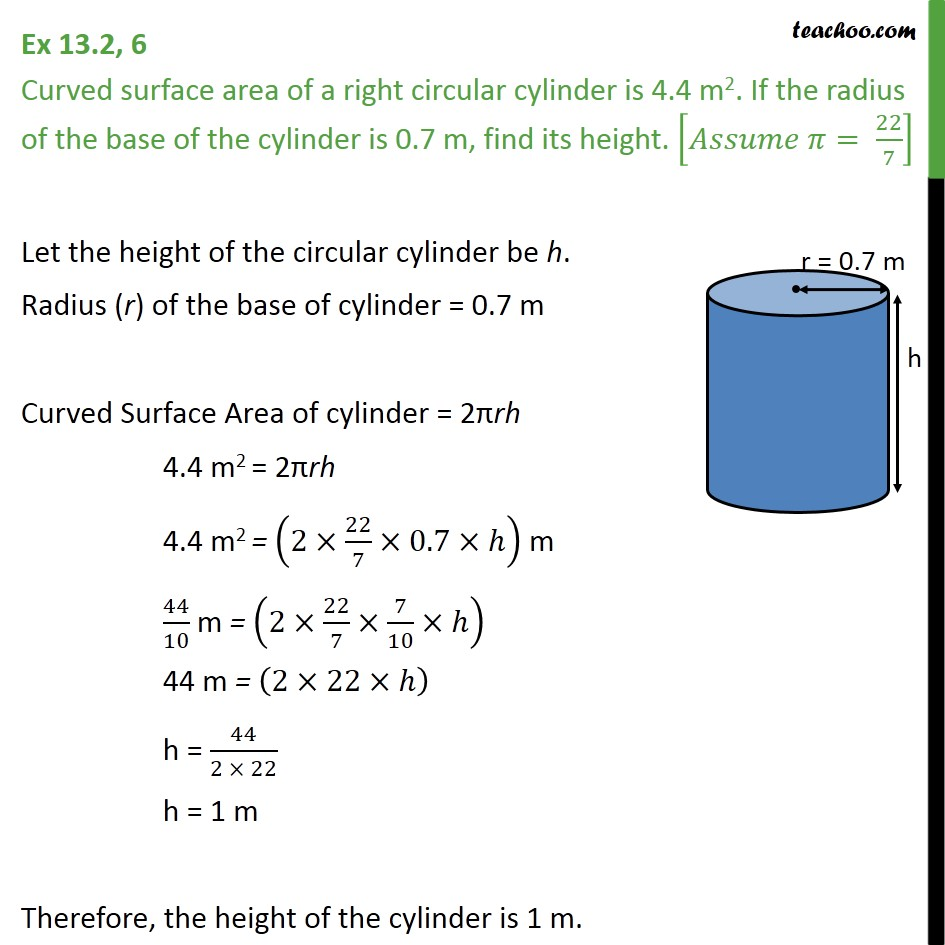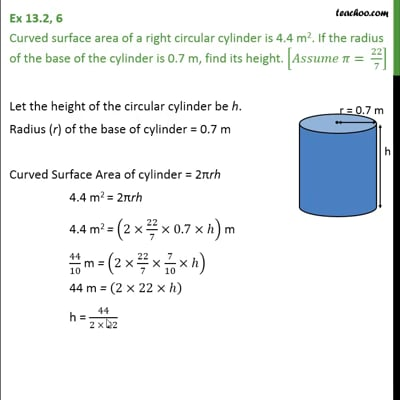Area Of Cylinder

Chapter 13 Class 9 Surface Areas and Volumes
Concept wiseThis video is only available for Teachoo black users

Introducing your new favourite teacher - Teachoo Black, at only ₹83 per month

### Transcript

Ex 13.2, 6 Curved surface area of a right circular cylinder is 4.4 m2. If the radius of the base of the cylinder is 0.7 m, find its height. [ = 22/7] Let the height of the circular cylinder be h. Radius (r) of the base of cylinder = 0.7 m Curved Surface Area of cylinder = 2 rh 4.4 m2 = 2 rh 4.4 m2 = (2 22/7 0.7 ) m 44/10 m = (2 22/7 7/10 ) 44 m = (2 22 ) h = 44/(2 22) h = 1 m Therefore, the height of the cylinder is 1 m.